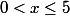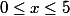### Day 34 - Extrema/Critical Numbers - 02.22.16

 Update New Seats!Summative Exam 1Average = Calculus Equations/Theorems What are extrema?extreme valuesminimamaximaall of the abovenone of the aboveWhat is the difference between relative and absolute extrema?Absolute extrema and relative extrema are always the same.Absolute extrema are based on the points that are either side of the extrema.Relative extrema are always the biggest or smallest values overall.Relative extrema can never be absolute extrema.none of the aboveWhich of the following represent the interval notation for the following: 0 < x < 5(0, 5)[0, 5)(0, 5][0, 5]none of the aboveWhich of the following represent the interval notation for the following:(0, 5)[0, 5)(0, 5][0, 5]none of the aboveWhich of the following represent the interval notation for the following:(0, 5)[0, 5)(0, 5][0, 5]none of the aboveReviewPre-calculusExtremaMinimaMaximaAbsolute vs. Relative ExtremaInterval NotationLessonObjectiveHow can extrema be defined for a function?How can critical numbers be calculated using derivatives?How are critical numbers related to extrema?Challenge 3.1Extreme Value TheoremSketch a graph that has no maxima or minima.Sketch a graph on an open interval that has no maxima or minima.Sketch a graph on a closed interval that has no maxima or minima.Sketch a graph on a closed interval that has a maxima, but no minima.Critical NumbersSketch a graph that has an extremum that is differentiable.Sketch a graph that has an extremum that is not differentiable.Sketch a graph that has a point in which the derivative is zero, but is not an extrema.VideosPracticeCheckpoints Exit Ticket Posted on the board at the end of the block.HomeworkStudy! Help Request List Standard(s) APC.7Analyze the derivative of a function as a function in itself.Includes:comparing corresponding characteristics of the graphs of f, f', and f''​defining the relationship between the increasing and decreasing behavior of f and the sign of f'​translating verbal descriptions into equations involving derivatives and vice versaanalyzing the geometric consequences of the Mean Value Theorem;defining the relationship between the concavity of f and the sign of f"; and ​identifying points of inflection as places where concavity changes and finding points of inflection.APC.8Apply the derivative to solve problems.Includes:​analysis of curves and the ideas of concavity and monotonicityoptimization involving global and local extrema;modeling of rates of change and related rates;use of implicit differentiation to find the derivative of an inverse function;interpretation of the derivative as a rate of change in applied contexts, including velocity, speed, and acceleration; anddifferentiation of nonlogarithmic functions, using the technique of logarithmic differentiation.**AP Calculus BC will also apply the derivative to solve problems.Includes:​analysis of planar curves given in parametric form, polar form, and vector form, including velocity and acceleration vectors;​numerical solution of differential equations, using Euler’s method;​l’Hopital’s Rule to test the convergence of improper integrals and series; and​geometric interpretation of differential equations via slope fields and the relationship between slope fields and the solution curves for the differential equations.